# Serialization in C++

## Serialization in C++ with Examples:

In this article, I am going to discuss Serialization in C++ with Examples. Please read our previous article where we discussed File Handling in C++ with Examples.

##### What is Serialization in C++?

Serialization is the process of storing and retrieving the state of an object. Suppose we want to store the student information in a file and again we want to retrieve the information of a student from a file. While working with Serialization the class must have a default constructor. For a better understanding, please have a look at the following example.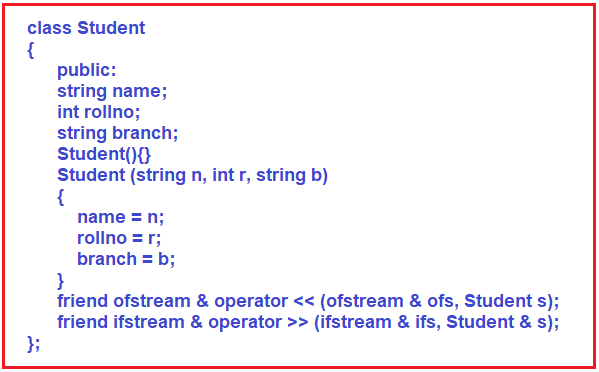Here we have a class Student which is having 3 members that are name, rollno, and branch. The rest of the things, we will explain later. Let us have a look at the main function: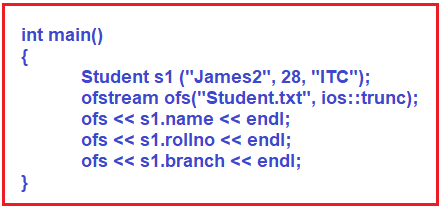Inside the main function, we have created an object s1 of class Student. So, once we have initialized the object, then we want to store this object in the file. In the previous article, we have seen how to store individual values but here we want to store this as an object.

Next, we have created a text file of the name “Student.txt” with ios::trunc mode so that everything will be removed from the file. We want to save all the object information in this Student.txt file output stream. So, we have to store name, roll no, and branch separately. So, after creating the file, we have stored all these things in the Student.txt inside the main function. The complete example code is given below.

```#include<iostream>
#include<fstream>
using namespace std;
class Student
{
public:
string name;
int rollno;
string branch;
Student ()
{
}
Student (string n, int r, string b)
{
name = n;
rollno = r;
branch = b;
}
friend ofstream & operator << (ofstream & ofs, Student s);
friend ifstream & operator >> (ifstream & ifs, Student & s);
};

int main()
{
Student s1 ("James", 28, "IT");
ofstream ofs ("Student.txt", ios::trunc);
ofs << s1.name << endl;
ofs << s1.rollno << endl;
ofs << s1.branch << endl;
}
```

Now, run the above code and you will see that the Student.txt file will be created in the location where you saved your project with the following content.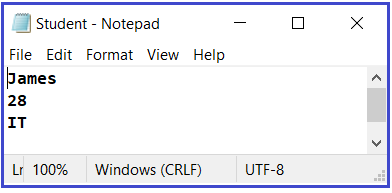Now let us understand Serialization in C++. Is it not possible that if we write?

ofs << s1;

And the complete object will be written on the file? Why we have stored all the values individually? If we can store the complete object with name, rollno and branch then we can say that we have stored the object. So, if we can store the whole object at once then we can store an object as well as we can retrieve the object. Yes. This is possible. So how it is possible? We have to overload the operator of the ofs stream in the student class. We have already discussed operator overloading in our previous articles.

##### Storing the state of an Object in a File:

So, we have defined some friend functions inside the Student class. The syntax of overloading is:

friend ofstream & operator << (ofstream & ofs, Student s);

We know well that when we want to override insertion or extraction operators then we must make them a friend function. Here ofstream is a file output stream, & means reference and we are overriding << operator that is insertion operator on file not on a console. Let us see the function body: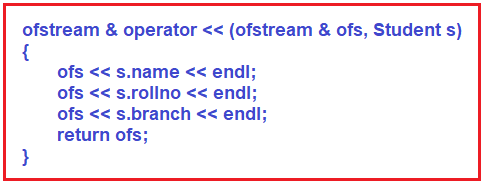Inside this function, we have written, ofs << s.name << endl. And same written for rollno and branch. And at the end, we have returned ofs that is output file stream. So that’s it. We have overloaded << operator.

So instead of writing individually for name, rollno, and branch, now we can write ofs << s1; inside the main function. This will write the entire data of the Student class object in the file. So, this is about storing the state of an object in a file. So main function is: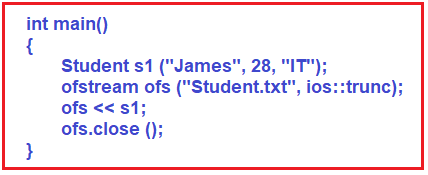Here the program becomes simple because the complexity of writing the contents of a class inside a file will be handled by the class itself with the help of a friend function by overloading an operator. Now, what is the main function is doing? The main function is creating an object for the student class, initializing it, and storing it. So, if you run this code then the Student.txt file will be created which contains the Student object’s data. So that’s all about storing the state of an object. The complete example code is given below.

```#include<iostream>
#include<fstream>
using namespace std;

class Student
{
public:
string name;
int rollno;
string branch;
Student ()
{
}
Student (string n, int r, string b)
{
name = n;
rollno = r;
branch = b;
}
friend ofstream & operator << (ofstream & ofs, Student s);
friend ifstream & operator >> (ifstream & ifs, Student & s);
};

ofstream & operator << (ofstream & ofs, Student s)
{
ofs << s.name << endl;
ofs << s.rollno << endl;
ofs << s.branch << endl;
return ofs;
}

int main()
{
Student s1 ("James", 28, "IT");
ofstream ofs ("Student.txt", ios::trunc);
ofs << s1;
ofs.close ();
}
```

Now, run the above code and you will see that the Student.txt file will be updated with the following content.Now let us look at how to retrieve the state of an object.

##### Retrieving the State of an Object in C++:

Let us see how to read the contents from the file.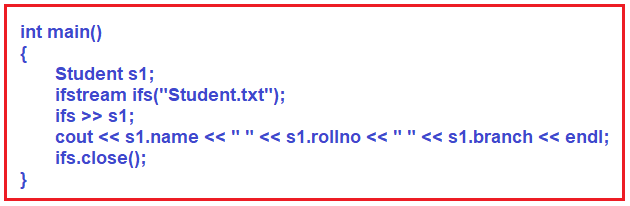Inside the main function, we have created an object of class Student. Then we have created an object ifs of ifstream that is the input file stream. Then we are printing the contents of s1. This will give an error as we haven’t overloaded the extraction operator in the Student class. So let us first overload the extraction operator that is >>. We have already written the signature for overload >> operator in Student class. So, the syntax is,

friend ifstream & operator >> (ifstream & ifs, Student & s);

Here we have ifstream instead of ofstream and >> instead of <<. Let us see a complete function.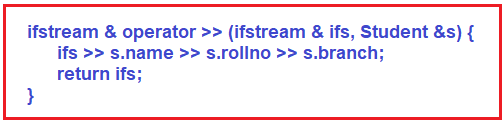In this function, we have read the values of name, rollno, and branch from the Student.txt file. And at last, we have returned the ifs object that is the input file stream. Make sure you have used the reference of the Student object in these functions. Otherwise, the object will not be updated. So here we have overloaded the extraction operator. Now we can write ifs >> s1 inside the main function. So, this will be retrieving the state of a student.

Now the data of the Student from the file is managed by these operators which are friends of the Student class. These operators are there which are handling everything how to write and read the data from the file.

##### Example to Understand Serialization in C++:
```#include<iostream>
#include<fstream>
using namespace std;

class Student
{
public:
string name;
int rollno;
string branch;
Student ()
{
}
Student (string n, int r, string b)
{
name = n;
rollno = r;
branch = b;
}
friend ofstream & operator << (ofstream & ofs, Student s);
friend ifstream & operator >> (ifstream & ifs, Student & s);
};

ofstream & operator << (ofstream & ofs, Student s)
{
ofs << s.name << endl;
ofs << s.rollno << endl;
ofs << s.branch << endl;
return ofs;
}

ifstream & operator >> (ifstream & ifs, Student & s)
{
ifs >> s.name >> s.rollno >> s.branch;
return ifs;
}

int main()
{
Student s1("James", 28, "IT");
ofstream ofs("Student.txt", ios::trunc);
ofs << s1;
ofs.close();

Student s2;
ifstream ifs("Student.txt");
ifs >> s2;
cout << s2.name << " " << s2.rollno << " " << s2.branch << endl;
ifs.close();
}
```

Output: James 28 IT

In the next article, I am going to discuss Working with Test and Binary Files in C++ with Examples. Here, in this article, I try to explain Serialization in C++ with Examples and I hope you enjoy this Serialization in C++ with Examples article. I would like to have your feedback. Please post your feedback, question, or comments about this Serialization in C++ with Examples article.